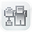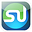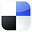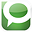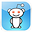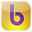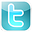Excel
STATISTICAL
Master

Confidence Intervals

Clear and Complete - WITH LOTS OF SOLVED PROBLEMS

# The Confidence Interval

Lots of Worked-Out, Easy-To-Understand, Graduate-Level Problems  --->
( Scroll Down and Take a Look ! )

Introduction

A Confidence Interval is an estimate of a population's average or proportion based upon sample data drawn from the population. A Confidence Interval is a range of values in which the mean is likely to fall with a specified level of confidence or certainty.

## Basic Explanation of Confidence Intervals

The Confidence Interval is an interval in which the true population mean or proportion probably lies based upon a much smaller random sample taken from that population.

Confidence Intervals for means are calculated differently than Confidence Intervals for proportions. The first half of this course module will discuss calculating a Confidence Interval for a population mean. The second half will cover calculating a Confidence Interval for a population proportion.

First we will briefly discuss the difference between sampling for mean and sampling for proportion:

## Mean Sampling vs. Proportion Sampling

What determines whether a mean is being estimated or a proportion is being estimated is the number of possible outcomes of each sample taken.

Proportion samples have only two possible outcomes.
For example, if you are comparing the proportion of Republicans in two different cities, each sample has only two possible values; the person sampled either is a Republican or is not.

Mean samples have multiple possible outcomes.
For example, if you are comparing the mean age of people in two different cities, each sample can have numerous values; the person sampled could be anywhere from 1 to 110 years old.

Below is a description of how to calculate a Confidence Interval for a population's mean. Note that everything is almost the same as the calculation of the Confidence Interval for a population proportion, except sample standard error.

## Confidence Interval of a Population Mean

The Confidence Interval of a Mean is an interval in which the true population mean probably lies based upon a much smaller random sample taken from that population.

A 95% Confidence Interval of a Mean is the interval that has a 95% chance of containing the true population mean.

The width of a Confidence Interval is affected by the sample size. The larger the sample size, the more accurate and tighter is the estimate of the true population mean. The larger the sample size, the smaller will be the Confidence Interval. Samples taken must be random and also be representative of the population.

### Calculate Confidence Intervals Using Large Samples(n>30)

Confidence Intervals are usually calculated and plotted on a Normal curve. If the sample size is less then 30, the population must be known to be Normally distributed. If small-sample data (n<30) is used to plot the Confidence Interval of the Mean for a population that is not Normally distributed, the result can be totally wrong.

Probably the most common major mistake in statistics is to apply Normal or t distribution tests to small-sample data taken from a population of unknown distribution. Typically the actual distribution of a population is not known.

If the population's underlying distribution is not known (usually it is not), then only large samples (n>30) are valid for creating a Confidence Interval of the Mean. The most important theorem of statistics, the Central Limit Theorem, explains the reason for this.

## The Central Limit Theorem

The Central Limit Theorem is statistics' most fundamental theorem. In a nutshell, it states the following: Random sample data can be plotted on a Normal curve to estimate a population's mean no matter how the population is distributed, as long as sample size is large (n>30).

The above definition of the Central Limit Theorem is the most practical and easy-to understand. The following definition of this theorem is a bit more technical and will satisfy statisticians (but basically says the same thing as the above): No matter how the population is distributed, the sampling distribution of the mean approaches the Normal curve as sample size becomes large.

## Levels of Confidence and Significance

Level of Significance, α ("alpha"), equals the maximum allowed percent of error. If the maximum allowed error is 5%, then α = 0.05.

Level of Confidence is the desired degree of certainty. A 95% Confidence Level is the most common. A 95% Confidence Level would correspond to a 95 Confidence Interval of the Mean. This would state that the actual population mean has a 95% probability of lying within the calculated interval. A 95% Confidence Level corresponds to a 5% Level of Significance, or α = 0.05. The Confidence Level therefore equals 1 - α.

## Population Mean vs. Sample Mean

Population Mean
= µ ("mu") (This is what we are trying to estimate)

Sample Mean = xavg

## Standard Deviation and Standard Error

Standard Deviation is a measure of statistical dispersion. Standard Deviation equals the square root of the Variance. It's formula is the following:

SQRT ( [ SUM (x - xavg)2 ] / N )

Population Standard Deviation = σ ("sigma")

Sample Standard Deviation = s

Standard Error is an estimate of population Standard Deviation from data taken from a sample. If the population Standard Deviation, σ, is known, then the Sample Standard Error, sxavg, can be calculated. If only the Sample Standard Deviation, s, is known, then Sample Standard Error, sxavg, can be estimated by substituting Sample Standard Deviation, s, for Population Standard Deviation, σ, as follows:

Sample Standard Error = sxavg = σ / SQRT(n) s / SQRT(n)

σ = Population standard deviation
s = Sample standard deviation
n = sample size

## Region of Certainty vs. Region of Uncertainty

Region of Certainty
is the area under the Normal curve that corresponds to the required Level of Confidence. If a 95% percent Level of Confidence is required, then the Region of Certainty will contain 95% of the area under the Normal curve. The outer boundaries of the Region of Certainty will be the outer boundaries of the Confidence Interval.

The Region of Certainty, and therefore the Confidence Interval, will be centered about the mean. Half of the Confidence Interval is on one side of the mean and half on the other side.

Region of Uncertainty is the area under the Normal curve that is outside of the Region of Certainty. Half of the Region of Uncertainty will exist in the right outer tail of the Normal curve and the other half in the left outer tail. This is similar to the concept of the "two-tailed test" that is used in Hypothesis testing in further sections of this course. The concepts of one- and two-tailed testing are not used when calculating Confidence Intervals. Just remember that the Region of Certainty, and therefore the Confidence Interval, are always centered about the mean on the Normal curve.

Relationship Between Region of Certainty, Uncertainty, and Alpha - The Region of Uncertainty corresponds to a ("alpha"). If a = 0.05, then that Region of Uncertainty contains 5% of the area under the Normal curve. Half of that area (2.5%) is in each outer tail. The 95% area centered about the mean will be the Region of Certainty. The outer boundaries of this Region of Certainty will be the outer boundaries of the 95% Confidence Interval. The Level of Confidence is 95% and the Level of Significance, or maximum error allowed, is 5%.

# The 95% Confidence Interval Illustrated With a Two-Tailed Normal Curve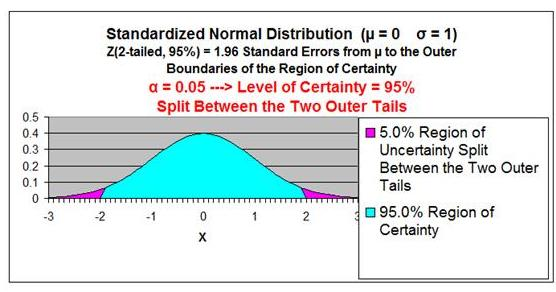Z Score Chart

Z Score at x (Inner Numbers - Yellow)
vs.
Area Under Normal Curve
Between Mean (µ) and x  (Outer Numbers - Green)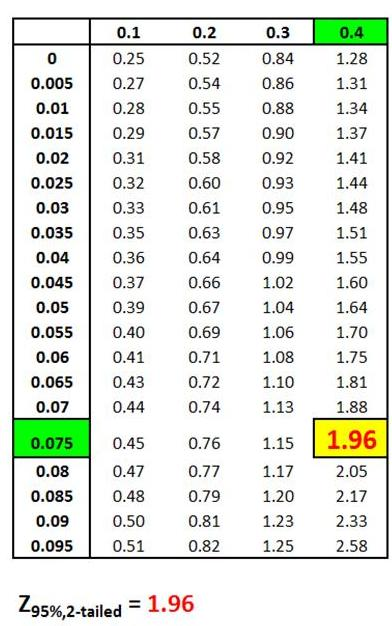# The 95% Confidence Interval Illustrated With a One-Tailed Normal Curve – Right Tail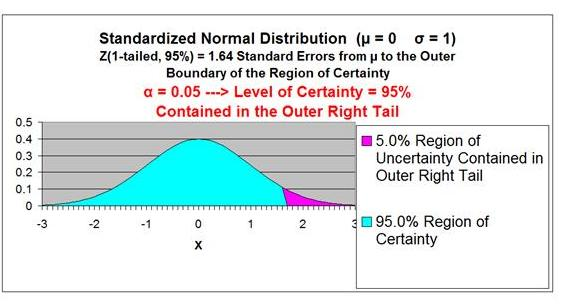Z Score Chart

Z Score at x (Inner Numbers - Yellow)
vs.
Area Under Normal Curve
Between Mean (µ) and x  (Outer Numbers - Green)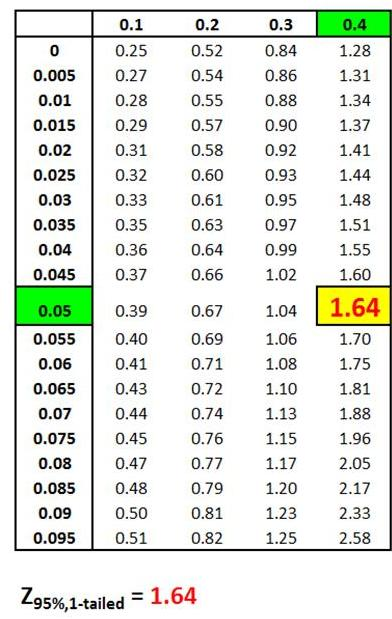## The 95% Confidence Interval Illustrated With a One-Tailed Normal Curve – Left TailZ Score Chart

Z Score at x (Inner Numbers - Yellow)
vs.
Area Under Normal Curve
Between Mean (µ) and x  (Outer Numbers - Green)## Z Score

Z Score is the number of Standard Errors from the mean to outer right boundary of the Region of Certainty (and therefore to the outer right boundary of the Confidence Interval). Standard Errors are used and not Standard Deviations because sample data is being used to calculate the Confidence Interval.

It is very important to note that on a Standardized Normal Curve, the Distance from the mean to boundary of the Region of Certainty equals the number of standard errors from the mean to boundary, which is the Z Score.

The above is only true for a Standardized Normal Curve. It is NOT true for a Non Standardized Normal curve.

Z Score(1 - α) = Number of Standard errors from mean to boundary of Confidence Interval. Note that (1 - α/2) = the entire area in the Normal curve to the left of outer right boundary of the Region of Certainty, or Confidence Interval. This includes the entire Region of Certainty and the half of the Region of Uncertainty that exists in the left tail.

For example:

Level of Confidence = 95% for a 95% Confidence Interval
Level of Significance (α)= 5%
Two-Tailed Curve

1 - α = 0.95 = 95%

Z Score(95% 2-Tailed) = 1.96

The outer right boundary of the 95% Confidence Interval, and the Region of Certainty, is 1.96 Standard Errors from the mean. The left boundary is the same distance from the mean because the Confidence Interval is centered about the mean.Z Score = 1.96

Z Score Chart

Z Score at x (Inner Numbers - Yellow)
vs.
Area Under Normal Curve
Between Mean (µ) and x  (Outer Numbers - Green)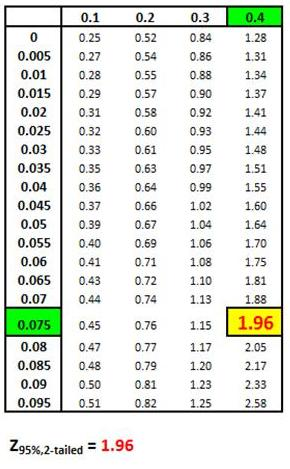The above table indicates that 95% of the area under the Normal curve lies within 1.96 Standard Errors of the mean if a two-tailed test is used. This indicates that 2.5% of the total area lies outside 1.96 Standard Errors of the mean on either side of the mean if a two-tailed test is used.

## Formulas for Calculating Confidence Interval Boundaries from Sample Data

The Confidence Interval is the width of the Region of Certainty. The Region of Certainty extends the same distance from the mean to the left and to the right because the Normal curve is symmetrical about the mean.

The Confidence Interval extends to the left and to the right of the mean by the following distance:

Z Score(1-α) * Sample Standard Error

which equals

Z Score(1-α) * sxavg

Therefore:

Confidence Interval Boundaries =
= Sample mean +/- Z Score(1-α) * Sample Standard Error

Confidence Interval Boundaries = xavg +/- Z Score(1-α) * sxavg

Confidence Interval Boundaries = xavg +/- Z Score(1-α) * σ / SQRT(n)

Confidence Interval Boundaries xavg +/- Z Score(1-α)) * s / SQRT(n)

As a result of:

Sample Mean = xavg

Sample Standard Deviation = s

Sample Standard Error = sxavg = σ / SQRT(n) s / SQRT(n)

Sample Size = n

For example, determine the 95% Confidence Interval if the Sample Size is 30, the Sample Standard Deviation = 3, the sample average  = 0, and the population from which the sample is drawn is Normally Distributed.

α - 1 - 95% =  1 - 0.95 = 0.05
Sample Standard Deviation
= s = 3
Sample Size = n = 30
Sample Mean = xavg = 0

Sample Standard Error = sxavg = σ / SQRT(n) s / SQRT(n) = 3 / SQRT(30)

Sample Standard Error
3 / SQRT(30)  =  0.5477

Z Score(1-α) = Z Score(95%, 2-tailed) = 1.96

Confidence Interval Boundaries = xavg +/- Z Score(1-α) * sxavg

Confidence Interval Boundaries xavg +/- Z Score(1-α) * s / SQRT(n)

Confidence Interval Boundaries
0 +/- (1.96) * (0.5477) =  0 +/- 1.074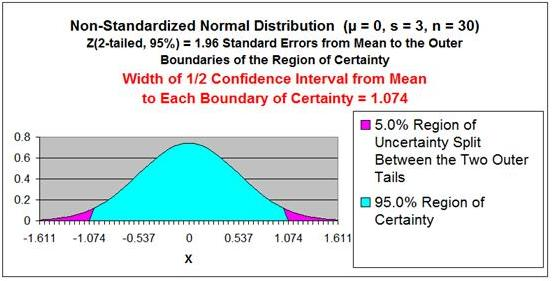Problem 1

Problem 1: Calculate a Confidence Interval from a Random Sample of Test Scores

Problem: Given the following set of 32 random test scores taken from a much larger population, calculate with 95% certainty an interval in which the population mean test score must fall. In other words, calculate the 95% Confidence Interval for the population test score mean. The random sample of 32 tests scores is shown below.

32 Random Test Score Samples from a Much Larger Population

 220 300 370 410 500 540 640 660 220 300 370 410 500 540 640 660 220 300 370 410 500 540 640 660 220 300 370 410 500 540 640 660

Level of Confidence = 95% = 1 - α

Level of Significance = α = 0.05

Sample Size = n = 32

Sample Standard Deviation = s = 149.5

Sample Standard Error = sxavg = σ / SQRT(n) s / SQRT(n) = 149.8 / SQRT(32)

Sample Standard Error
149.8 / SQRT(32)  =  26.5

Z Score(1-α) = Z Score(95%, 2-tailed) = 1.96

Confidence Interval Boundaries = xavg +/- Z Score(1-α) * sxavg

Confidence Interval Boundaries xavg +/- Z Score(1-α) * s / SQRT(n)

Confidence Interval Boundaries
455 +/- (1.96) * (26.5) =  455 +/- 51.9

Confidence Interval Boundaries 403.1  to  506.9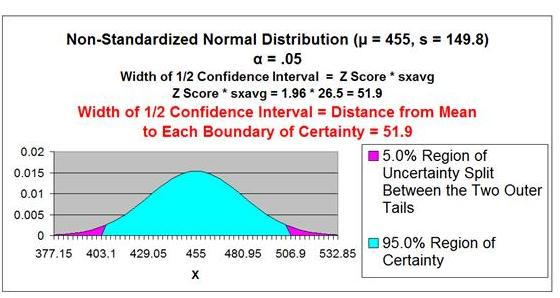Z Score Chart

Z Score at x (Inner Numbers - Yellow)
vs.
Area Under Normal Curve
Between Mean (µ) and x  (Outer Numbers - Green)This same problem above is solved in the Excel Statistical Master with only 3 Excel formulas (and NO looking anything up on a Z Chart). Everything is explained to you in SIMPLE language in the Excel Statistical Master.

Problem 2

Problem 2: Calculate a Confidence Interval of Daily Sales Based Upon Sample Mean and Standard Deviation

Problem: Average daily demand for books sold in a small Barnes and Noble store is 455 books with a standard deviation of 200. This average and standard deviation are taken from sale data collected every day for a period of 60 days. What is the range that the true average daily book sales lies in with 95% certainty?

Level of Confidence = 95% = 1 - α

Level of Significance = α = 0.05

Sample Size = n = 60

Sample Mean = xavg = 455

Sample Standard Deviation = s = 200

Sample Standard Error = sxavg = σ / SQRT(n) s / SQRT(n)

sxavg = σ / SQRT(n) s / SQRT(n) = 200 / SQRT(60) = 25.8

Z Score(1 - α) = Z Score(95% 2-Tailed) = 1.96

Width of Half the Confidence Interval = Z Score(1-α) * sxavg

Width of Half the Confidence Interval = Z Score(1-α) * σ / SQRT(n)

Width of Half the Confidence Interval xavg +/- Z Score(1-α) * s / SQRT(n)

= 1.96 * 25.8 = 50.6

Confidence Interval Boundaries = xavg +/- Z Score(1-α) * sxavg

455 +/- (1.96)*(25.8) = 455 +/- 50.6 = 404.4 to 505.6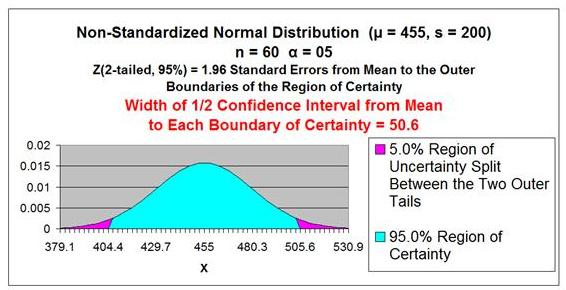This same problem above is solved in the Excel Statistical Master with only 3 Excel formulas (and not having to look anything up on a Z Chart). The Excel Statistical Master teaches you everything in step-by-step frameworks. You'll never have to memorize any complicated statisical theory.

Problem 3

Problem 3: Calculate an Exact Range of 95% of Sales Based Upon the Upon the Population Mean and Standard Deviation

Problem: Average daily demand for books sold in a large Barnes and Noble store is 5,000 books with a standard deviation of 200. This average and standard deviation are taken from sale data collected every day for a period of 5 years. What is the range that 95% of the daily unit book sales falls in? The daily sales data is Normally distributed.

This problem is not a Confidence Interval problem. We do not need to create an estimate of the population mean (a Confidence Interval) because we know exactly what it is. We are given the population mean and population standard deviation.

We do need to know how the population is distributed in order to calculate the interval that contains 95% of all population data. Given that the population is Normally distributed, we simply need to map the region of this Normal curve that contains 95% of the total area and is centered about the mean as follows:

Population mean = µ = 5,000

Population Standard Deviation = σ = 200

Range Containing 95% of Sales Data = µ +/- [ Z Score(95% 2-Tailed) ]  * σ  ]

= 5,000 +/- [ 1.96 * 200 ]

= 5,000 +/- 392

= 4,608 to 5,392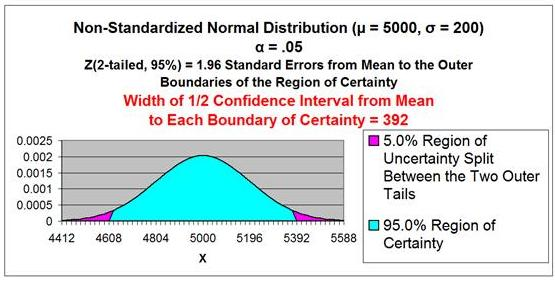Z Score Chart

Z Score at x (Inner Numbers - Yellow)
vs.
Area Under Normal Curve
Between Mean (µ) and x  (Outer Numbers - Green)This same problem above is solved in the Excel Statistical Master with only 1 Quick Excel formula (and NO looking anything up on a Z Chart). You'll be able to grasp your statistics course a LOT easier with the Excel Statistical Master.

## Determining Minimum Sample Size (n) to Keep Confidence Interval of the Mean within a Certain Tolerance

The larger the sample size, the more accurate and tighter will be the prediction of a population's mean. Stated another way, the larger the sample size, the smaller will be the Confidence Interval of the population's mean. Width of the Confidence Interval is reduced when sample size is increased.

Quite often a population's mean needs to be estimated with some level of certainty to within plus or minus a specified tolerance. This specified tolerance is half the width of the Confidence Interval. Sample size directly affects the width of the Confidence Interval. The relationship between sample size and width of the Confidence Interval is shown as follows:

n = Min Sample Size Required to Keep Confidence Interval Within a
Required Tolerance for a Given Confidence Level and
Standard Deviation

Width of Half of the Confidence Interval = Z Score(1-α) * σ / SQRT(n)

Width of Half of the Confidence Interval Z Score(1-α) * sxavg

As a result of:

Z Score(1-α) * σ / SQRT(n)      Z Score(1-α) * s / SQRT(n)

With algebraic manipulation of the above we have:

SQRT(n) = Z Score(1-α) * σ / Width of Half of the Confidence Interval

n = [ Z Score(1-α) ]2 * [σ]2 / [ Width of Half of the Confidence Interval ]2

Also, if we only have Sample Standard Deviation, s, and not Population
Standard Deviation, σ :

n [ Z Score(1-α) ]2 * [s]2 / [ Width of Half of the Confidence Interval ]2

Problem 4

Problem 4: Determine the Minimum Number of Sales Territories to Sample In Order To Limit the 95% Confidence Interval to a Certain Width

Problem: A national sales manager in charge of 5,000 similar territories ran a nationwide promotion. He then collected sales data from a random sample of the  territories to evaluate sales increase. From the sample, the average sales increase per territory was \$10,000 with a standard deviation of \$500. How many territories would he have had to have sampled to be 95% sure that the actual nationwide average territory sales increase was no more than \$50 different than average territory sales increase from the sample he took?

Level of Confidence = 95% = 1 - α

Level of Significance = α = 0.05

Sample Size = n = ?

n = Min Sample Size Required to Keep Confidence Interval Within a
Required Tolerance for a Given Confidence Level and
Standard Deviation

Sample Mean = xavg ------> Note this does not need to be known to solve this problem

Sample Standard Deviation = s = 500

Z Score(1 - α) = Z Score(95% 2-Tailed) = 1.96

Width of Half the Confidence Interval = 50

n [ Z Score(1-a) ]2 * [s]2 / [ Width of Half of the Confidence Interval ]2

n [ 1.96 ]2 * 2 / [ 50 ]2 = 384

The sales manager would have to sample at least 384 territories to be 95% certain that nationwide territory average was within +/- \$50 of the sample territory average. Note that the 95% confidence interval is \$10,000 +/- \$50 and this interval has a width = \$100 if sample size is 384.

This same problem above is solved in the Excel Statistical Master with only 2 Excel formulas (and not having to look anything up on a Z Chart). If you found your statistics book confusing, You'll really like the Excel Statistical Master. Everything is explained in simple, step-by-step frameworks.

## Confidence Interval of a Population Proportion

Creating a Confidence Interval for a population's proportion is very similar to creating a Confidence Interval for a population's mean. The only real difference is how the standard error is calculated. Everything else is the same. The method of calculating a Confidence Interval for a population mean was covered in  detail earlier in this module. First, the difference between using sampling to estimate a population mean and using sampling to estimate a population proportion will be explained below:

## Mean Sampling vs. Proportion Sampling

What determines whether a mean is being estimated or a proportion is being estimated is the number of possible outcomes of each sample taken.

Proportion samples have only two possible outcomes. For example, if you are comparing the proportion of Republicans in two different cities, each sample has only two possible values; the person sampled either is a Republican or is not.

Mean samples have multiple possible outcomes. For example, if you are comparing the mean age of people in two different cities, each sample can have numerous values; the person sampled could be anywhere from 1 to 110 years old.

Below is a description of how to calculate a Confidence Interval for a population's proportion. Note that everything is almost the same as the calculation of the Confidence Interval for a mean, except sample standard error.

## Levels of Confidence and Significance

Level of Significance, α ("alpha"), equals the maximum allowed percent of error. If the maximum allowed error is 5%, then α = 0.05.

Level of Confidence is selected by the user. A 95% Level is the most common. A 95% Confidence Level would correspond to a 95% Confidence Interval of the Proportion. This would state that the actual population Proportion has a 95%probability of lying within the calculated interval. A 95% Confidence Level corresponds to a 5% Level of Significance, or α, = 0.05. The Confidence Level therefore equals 1 - α.

## Population Proportion vs. Sample Proportion

Population Proportion = µp = p (This is what we are trying to estimate)

Sample Proportion = pavg

## Standard Deviation and Standard Error

Standard Deviation is not calculated during the creation of Confidence Interval for a population proportion.

Standard Error is an estimate of population Standard Deviation from data taken from a sample. Sample Standard Error will be an estimate taken from the sample proportion, pavg, and sample size, n. This is the major difference between calculating a Confidence Interval for a proportion and for a mean. Binomial distribution rules apply to proportions because a proportion sample has only two possible outcomes, just like a binomial variable.

Sample Standard Error of a Proportion = σpavg
σpavg = SQRT(p * q / n) spavg

Estimated Sample Standard Error of a Proportion = spavg
spavg = SQRT ( pavg * qavg / n )

p = Population proportion - This is the unknown that will be estimated with a Confidence Interval

q = 1 - p

n = Sample Size

pavg = Sample Proportion

qavg = 1 - pavg

## Region of Certainty vs. Region of Uncertainty

Region of Certainty is the area under the Normal curve that corresponds to the required Level of Confidence. If a 95% percent Level of Confidence is required, then the Region of Certainty will contain 95% of the area under the Normal curve. The outer boundaries of the Region of Certainty will be the outer boundaries of the Confidence Interval.

The Region of Certainty, and therefore the Confidence Interval, will be centered about the mean. Half of the Confidence Interval is on one side of the mean and  half on the other side.

Region of Uncertainty is the area under the Normal curve that is outside of the Region of Certainty. Half of the Region of Uncertainty will exist in the right outer tail of the Normal curve and the other half in the left outer tail. This is similar to the concept of the "two-tailed test" that is used in Hypothesis testing in further sections of this course. The concepts of one and two-tailed testing are not used when calculating Confidence Intervals. Just remember that the Region of Certainty, and therefore the Confidence Interval, are always centered about the mean on the Normal curve.

Relationship Between Region of Certainty, Uncertainty, and Alpha - The Region of Uncertainty corresponds to a ("alpha"). If α = 0.05, then that Region of Uncertainty contains 5%  of the area under the Normal curve. Half of that area (2.5%) is in each outer tail. The 95% area centered  about the mean will be the Region of Certainty. The outer boundaries of this Region of Certainty will be the outer boundaries of the 95% Confidence Interval. The Level of Confidence is 95% and the Level of Significance, or maximum error allowed, is 5%.

## Z Score

Z Score is the number of Standard Errors from the mean to outer right boundary of the Region of Certainty (and therefore to the outer right boundary of the Confidence Interval). Standard Errors are used and not Standard Deviations because sample data is being used to calculate the Confidence Interval.

It is very important to note that on a Standardized Normal Curve, the Distance from the mean to boundary of the Region of Certainty equals the number of standard errors from the mean to boundary, which is the Z Score.

The above is only true for a Standardized Normal Curve. It is NOT true for a Non Standardized Normal curve.

Z Score(1 - α) = Number of Standard errors from mean to boundary of Confidence Interval. Note that (1 - α/2) = the entire area in the Normal curve to the left of outer right boundary of the Region of Certainty, or Confidence Interval. This includes the entire Region of Certainty and the half of the Region of Uncertainty that exists in the left tail.

For example:

Level of Confidence = 95% for a 95% Confidence Interval
Level of Significance (α)= 5%
Two-Tailed Curve

1 - α = 0.95 = 95%

Z Score(95% 2-Tailed) = 1.96

The outer right boundary of the 95% Confidence Interval, and the Region of Certainty, is 1.96 Standard Errors from the mean. The left boundary is the same distance from the mean because the Confidence Interval is centered about the mean.Z Score = 1.96

Z Score Chart

Z Score at x (Inner Numbers - Yellow)
vs.
Area Under Normal Curve
Between Mean (µ) and x  (Outer Numbers - Green)The above table indicates that 95% of the area under the Normal curve lies within 1.96 Standard Errors of the mean if a two-tailed test is used. This indicates that 5% of the total area lies outside 1.96 Standard Errors of the mean in either side of the mean if a two-tailed test is used.

## Formula for Calculating Confidence Interval Boundaries from Sample Data for a Population Proportion

Confidence Interval Boundaries
= Sample proportion +/- Z Score(1-α) * Sample Standard Error

Confidence Interval Boundaries
= pavg +/- Z Score(1-α) * spavg

Sample Proportion = pavg

Sample Standard Error of a Proportion = σpavg spavg

Sample Standard Error of a Proportion = SQRT ( pavg * qavg / n )

Sample size = n

Confidence Interval Boundaries = pavg +/- Z Score(1-α) * spavg

Problem 5

Problem 5: Determine Confidence Interval of Shoppers Who Prefer to Pay By Credit Card Based Upon Sample Data

Problem: A random sample of 1,000 shoppers was taken. 70% preferred to pay with a credit card. 30% preferred to pay with cash. Determine the 95% Confidence Interval for the proportion of the general population that prefers to pay with a credit card.

Level of Confidence = 95% = 1 - α

Level of Significance = α = 0.05

Sample Size = n = 1,000

Sample Proportion = pavg = 0.70

qavg = 1 - pavg = 0.30

Sample Standard Error of a Proportion = σpavg spavg
spavg = SQRT ( pavg * qavg / n )

spavg = SQRT ( 0.70 * 0.30 / 1,000 ) = 0.014

Z Score(1 - α) = Z Score95% = 1.96

Width of Half the Confidence Interval = Z Score(1-α) * spavg

= 1.96 * 0.014 = 0.0274

Confidence Interval Boundaries = pavg +/- Z Score(1-a) * spavg

= 0.70 +/- (1.96) * (0.014)

= 0.70 +/- 0.0274 = 0.6726 to 0.7274 = 67.26% to 72.74%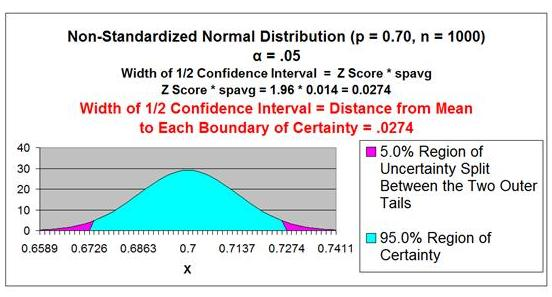This same problem above is solved in the Excel Statistical Master with only 2 Excel formulas. With the Excel Statistical Master you can do advanced business statistics without having to buy and learn expensive, complicated statistical software packages such as SyStat, MiniTab, SPSS, or SAS.

## Determining Minimum Sample Size (n) to Keep Confidence Interval of the Proportion within a Certain Tolerance

The larger the sample size, the more accurate and tighter will be the prediction of a population's mean. Stated another way, the larger the sample size, the smaller will be the Confidence Interval of the population's mean. Width of the Confidence Interval is reduced when sample size is increased.

Quite often a population's mean needs to be estimated with some level of certainty to within plus or minus a specified tolerance. This specified tolerance is half the width of the Confidence interval. Sample size directly affects the width of the Confidence Interval. The relationship between sample size and width of the Confidence Interval is shown as follows:

Width of Half the Confidence Interval =Z Score(1-α)* spavg

spavg = SQRT( pavg* qavg/ n)

Width of Half the Confidence Interval = Z Score(1-α)*SQRT( pavg* qavg/ n)

[ Width of Half the Confidence Interval ]2 =[Z Score(1-α) ]2*( pavg* qavg/ n)

n = [ Z Score(1-α)]2 * ( pavg * qavg ) / [ Width of Half the Confidence Interval ]2

Problem 6: Determine the Minimum Sample Size of Voters to be 95% Certain that the Population Proportion is no more than 1% Different from Sample Proportion.

Problem: A random survey was conducted in one city to learn voting preferences. 40% of voters surveyed said they would vote Republican. 60% of the voters surveyed said they would vote Democrat. Determine the minimum number of voters that had to be surveyed to be 95% certain that the results were accurate within
+/- 1%.

Level of Confidence = 95% = 1 - α

Level of Significance = α = 0.05

pavg = 0.40

qavg = 1 - pavg = 0.60

Width of Half the Confidence Interval = 0.01 ---> (1%)

Z Score(1 - a) = Z Score95% = 1.96

n = [ Z Score(1-α) ]2 * ( pavg * qavg ) / [ Width of Half the Confidence Interval ]2

n = [ 1.96 ]2 * ( 0.40 * 0.60 ) / [ 0.01 ]2 = 9,220

At least 9,220 random voters had to be surveyed to be 95% certain that the population proportion is no more than 1% different from the sample.

This same problem above is solved in the Excel Statistical Master with only 2 Excel formulas. The Excel Statistical Master is for you if you want to know how to apply statistics to solve real world business problems.

If You Like This, Then Share It...